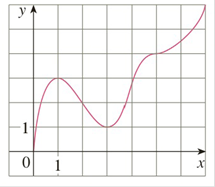Chapter 3.3, Problem 2E

Chapter
Section
Textbook Problem

# 1-2 Use the given graph of f to find the following.(a) The open intervals on which f is increasing.(b) The open intervals on which f is decreasing.(c) The open intervals on which f is concave upward.(d) The open intervals on which f is concave downward.(e) The coordinates of the points of inflection.To determine

(a)

To find:

The open interval on which f  is increasing.

Explanation

1) Concept:

From the graph, find the interval in which f(x) is increasing.

2) Calculation:

From the graph, the values of f(x) are increasing when x is increasing from 0 to 1 and 3to 7.

Therefore f(x)  is increasing in the intervals (0, 1

To determine

(b)

To find:

The open interval on which f  is decreasing.

To determine

(c)

To find:

The open intervals on which f is concave upward.

To determine

(d)

To find:

The open intervals on which f is concave downward.

To determine

(e)

To find:

Coordinates of points of inflection.

### Still sussing out bartleby?

Check out a sample textbook solution.

See a sample solution

#### The Solution to Your Study Problems

Bartleby provides explanations to thousands of textbook problems written by our experts, many with advanced degrees!

Get Started

#### Proof prove that cos(sin1x)=1x2

Calculus: Early Transcendental Functions

#### Finding Intercepts In Exercises 19-28, find any intercepts. x2yx2+4y=0

Calculus: Early Transcendental Functions (MindTap Course List)

#### In Exercises 724, compute the indicated products. 

Finite Mathematics for the Managerial, Life, and Social Sciences